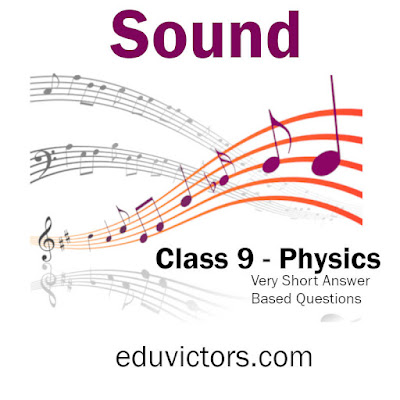# CBSE Class 9 Physics - Sound - Very Short Answer-Based Questions (VSQA)

Q1. Define vibration?

Answer: Rapid to and fro motion of an object.

Q2. How is sound produced?

Q3. Define wave.

Answer: A wave is a disturbance produced in a medium as the particles of the medium vibrate.

Q4. Is sound a type of wave?

Q5. Why sound is called mater wave?

Answer: Because it requires a medium to travel.

Q6. When a sound travels from speaker to listener through the air, do air particles also move from speaker to listener carrying sound energy?

Answer: No, the air particles vibrate at their mean positions. These vibrations form compressions and rarefactions (high-pressure and low-pressure regions). Sound energy moves through vibrating particles.

Q7. Name the physical quantity which determines the pitch of the sound.

Q8. A sound wave travels at a speed of 399 m/s. If its wavelength is 1.5 m, what is the wave's frequency? Will it be audible to us?

399 = υ × 1.5

υ =399/1.5 = 266 Hz

It will be audible

Q9. What is reverberation?

Answer: Reverberation is the ‘repeated reflection of sound waves that result in persistence of sound for some time.

Q10. What is the audible range of the human ear?

Answer: Between 20 Hz to 20,000 Hz.

Q11. Define loudness. On which physical quantity does it depend? What is the SI unit of loudness?

Answer: It is a measure of the sound energy reaching the ear per second.

It depends on the amplitude of the sound wave.

It is measured in decibels ‘dB’.

Q12. What is the difference between a tone and a note?

Answer: Tone: A pure sound of a single frequency is called tone.

Note: An impure sound produced by a mixture of many frequencies is called a note. For example, A musical note has tones of various frequencies.

Q13. What is the distance between a crest and the next trough in a transverse wave?

Answer: The distance between a crest and the next trough is half the wavelength i.e., λ/2.

Q14. What does SONAR stand for?

Q15. Why can't we hear an echo in a small room?

Answer: For an echo to be heard, the minimum distance between the source and the walls of the room should be 17.2m. That's in a small room (distance is much less than 17.2 m) echoes can't be heard.